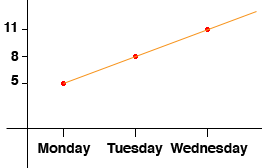SEARCH HOMEMath Central Quandaries & QueriesQuestion from Rebecca, a student: What is the definition of linear growth?Hi Rebecca,

Linear growth means that it grows by the same amount in each time step. For example you might have something that is 5 inches long on Monday morning and then 8 inches long on Tuesday morning and then 11 inches long on Wednesday morning and so on. So it is growing by 3 inches a day. If you draw a graph with the days on the horizontal axis and the height in inches on the vertical axis then the points lie on a line. This is why it is called linear growth.Some growth is not linear. If you have a checker board and put 1 penny on the first square, a stack of 2 pennies on the second square, a stack of 4 pennies on the third square, a stack of 8 pennies on the fourth square, that is you double the number of pennies in the stack at each step, then the growth is not linear.

PennyMath Central is supported by the University of Regina and The Pacific Institute for the Mathematical Sciences.